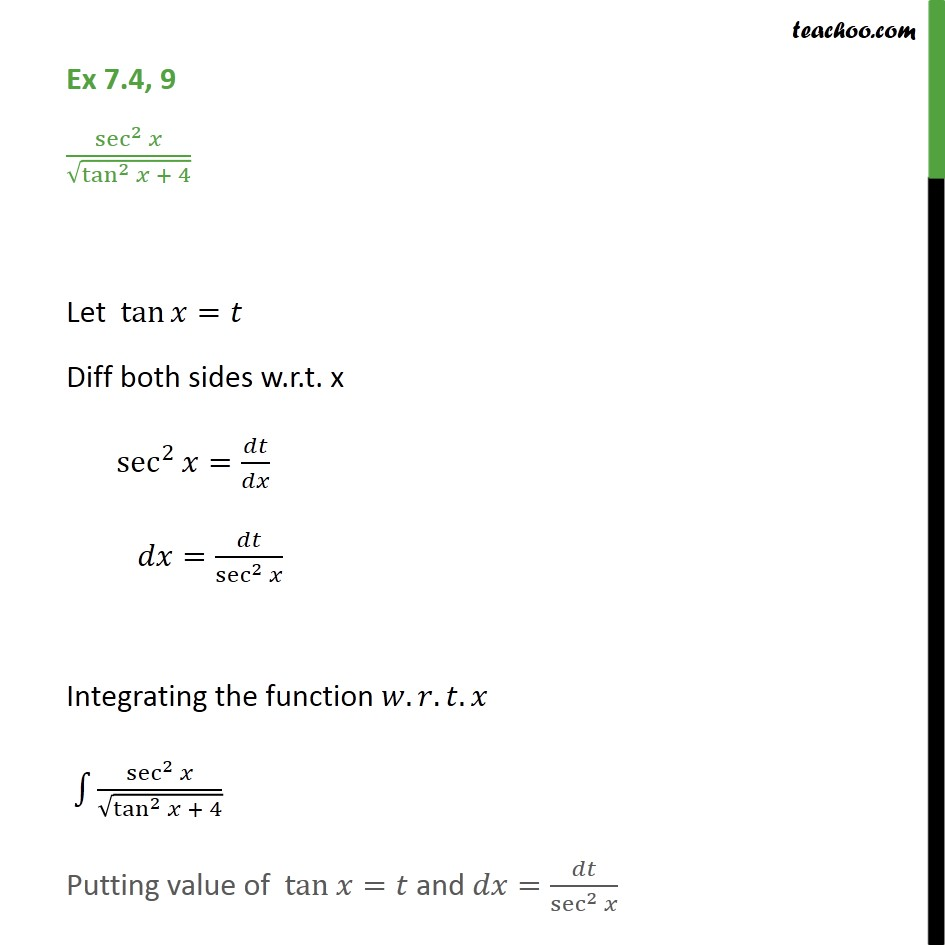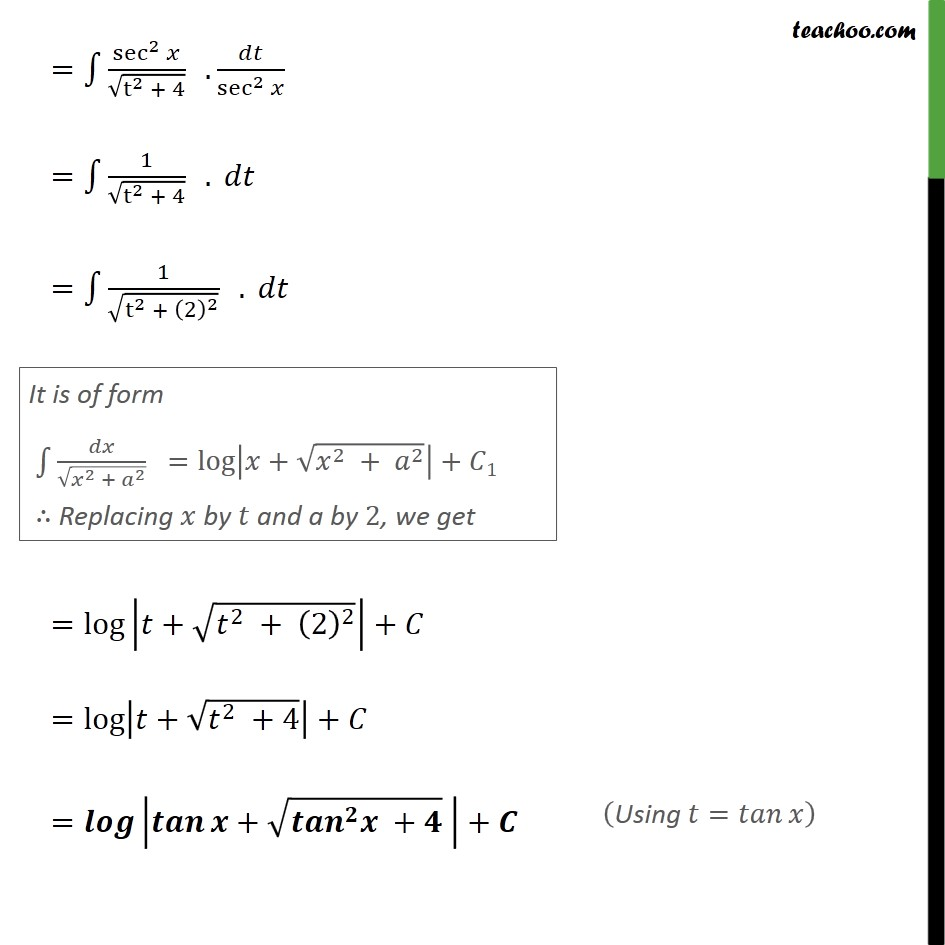Ex 7.4

Chapter 7 Class 12 Integrals
Serial order wiseLearn in your speed, with individual attention - Teachoo Maths 1-on-1 Class

### Transcript

Ex 7.4, 9 sec 2 tan 2 + 4 Let tan = Diff both sides w.r.t. x sec 2 = = sec 2 Integrating the function . . . sec 2 tan 2 + 4 Putting value of tan = and = sec 2 = sec 2 t 2 + 4 . sec 2 = 1 t 2 + 4 . = 1 t 2 + 2 2 . = log + 2 + 2 2 + = log + 2 +4 + = + + +